# CliquePercolation

#### 2022-11-09

The CliquePercolation package entails a number of functions related to the clique percolation community detection algorithms for undirected, unweighted and weighted networks (Farkas, Ábel, Palla, & Vicsek, 2007; as described in Palla, Derényi, Farkas, & Vicsek, 2005).

The package entails functions for…

• helping to optimize parameters of the algorithms
• running the algorithms
• plotting the results.

This document provides an introduction to this workflow with some random example data sets.

## An Introduction to the Clique Percolation Community Detection Algorithm

Interconnected entities can be represented as networks (Barabási, 2011). Each network entails two sets, namely nodes, which are the entities, and edges, which are the connections between entities. Many networks are undirected such that edges simply connect two nodes with each other without any directionality in the edges. For instance, nodes could represent people and edges could represent whether they are friends or not. Or nodes could represent cities and edges could represent whether there is a street connecting the cities. Such networks, in which edges are either present or absent, are called unweighted. In other cases, nodes could represent people and edges could represent the frequency with which these people meet. Or nodes could represent questionnaire items and edges could represent the correlations of these items. In such a case, the edges are not only present or absent, but may count the frequency or strength of interactions. Such networks are called weighted. The edges in the network can further be directed, such that edges can point from one node to another. For instance, the internet is a network of webpages in which a directed edge could represent a hyperlink from one webpage to another. Here, only undirected networks are discussed.

Analyzing the structure of such networks is a key task across sciences. One structural feature that is often investigated is the identification of strongly connected subgraphs in the network, which is called community detection (Fortunato, 2010). Most community detection algorithms thereby put each node in only one community. However, it is likely that nodes are often shared by a number of communities. This could occur, for instance, when a friend is part of multiple groups. One community detection algorithm that is aimed at identifying overlapping communities is the clique percolation algorithm, which has been developed for unweighted (Palla et al., 2005) and weighted networks (Farkas et al., 2007).

The clique percolation algorithm for unweighted networks proceeds as follows:

• First, you identify a network of nodes and edges. I will use an unweighted network with eight nodes.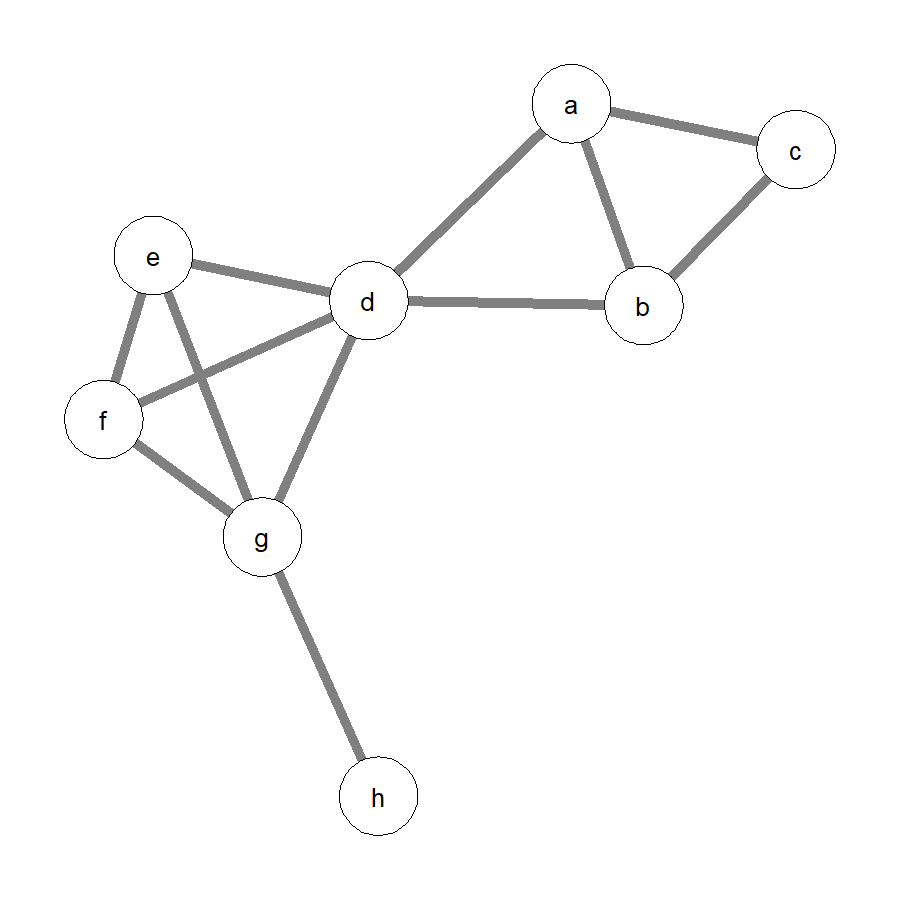Unweighted network with eight nodes.

• Second, you identify k-cliques, which are fully connected networks with $$k$$ nodes. The smallest possible $$k$$ would be $$k = 3$$. Otherwise, the cliques would be only edges. Applying $$k = 3$$ to the example leads to the identification of six 3-cliques, namely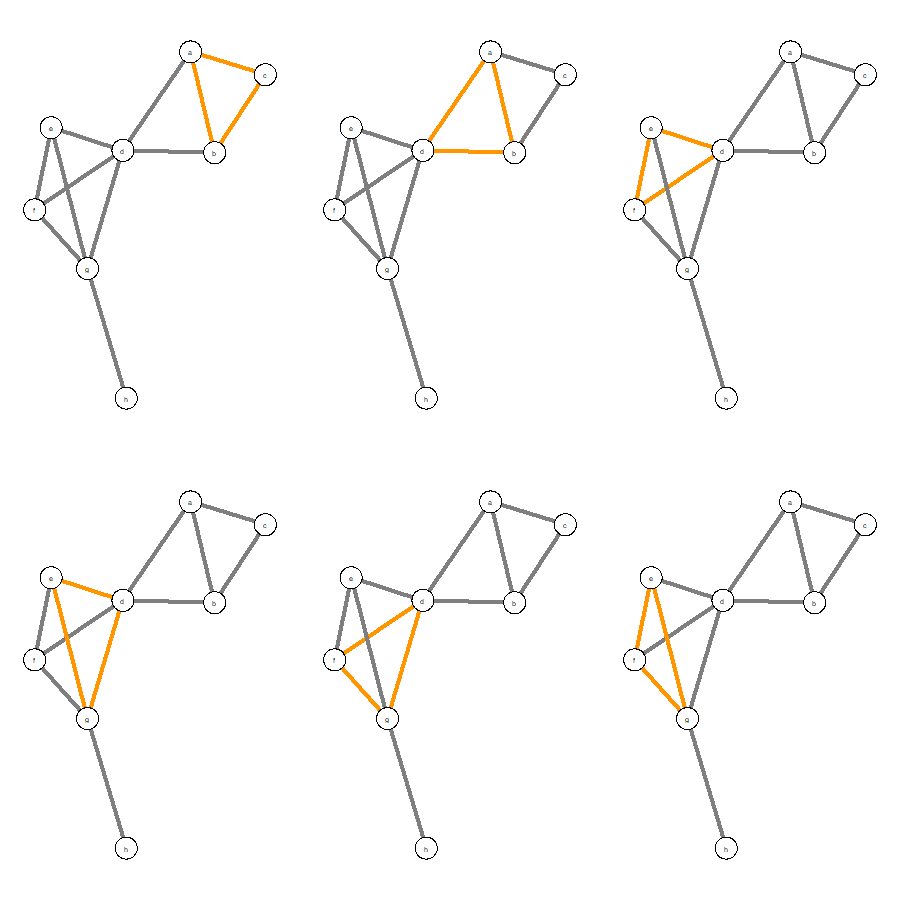Six 3-cliques in unweighted network.

• Finally, a community is defined as a set of adjacent $$k$$-cliques, that is, $$k$$-cliques that share exactly $$k-1$$ nodes. With $$k = 3$$, two 3-cliques are adjacent if they share exactly two nodes (equivalent to an edge). In the example, this implies that there are two communities (see below). The green edges entail the 3-cliques $$a$$$$b$$$$c$$ and $$a$$$$b$$$$d$$. The pink edges entail the 3-cliques $$d$$$$e$$$$f$$, $$d$$$$e$$$$g$$, $$d$$$$f$$$$g$$, and $$e$$$$f$$$$g$$.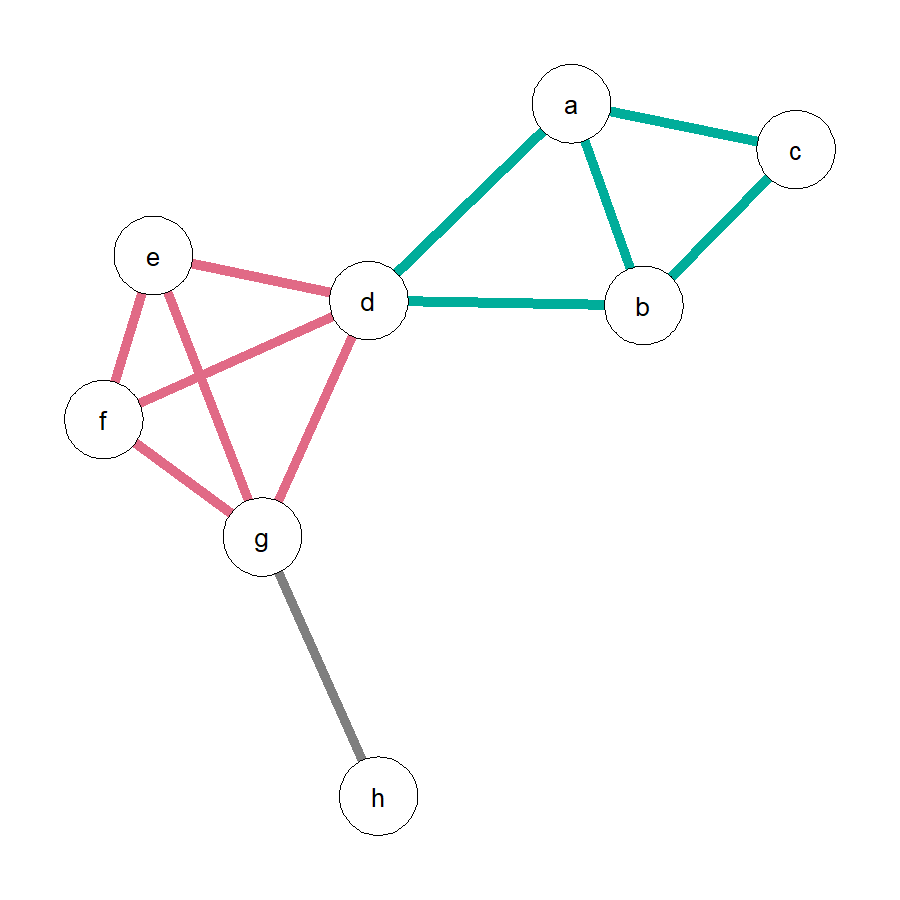Two communities in unweighted network.

This also showcases that the clique percolation algorithm can lead to nodes that are shared by communities. In the current example, node $$d$$ is part of both the green and the pink community. Nodes $$a$$, $$b$$, and $$c$$ are part of only the green community and nodes $$e$$, $$f$$, and $$g$$ are part of only the pink community. Importantly, clique percolation can also lead to nodes that are part of no community. These are called isolated nodes, in the current example, node $$h$$.

One way to plot the community partition on the original network would be to color the nodes according to the communities they belong to. Shared nodes have multiple colors and isolated nodes remain white.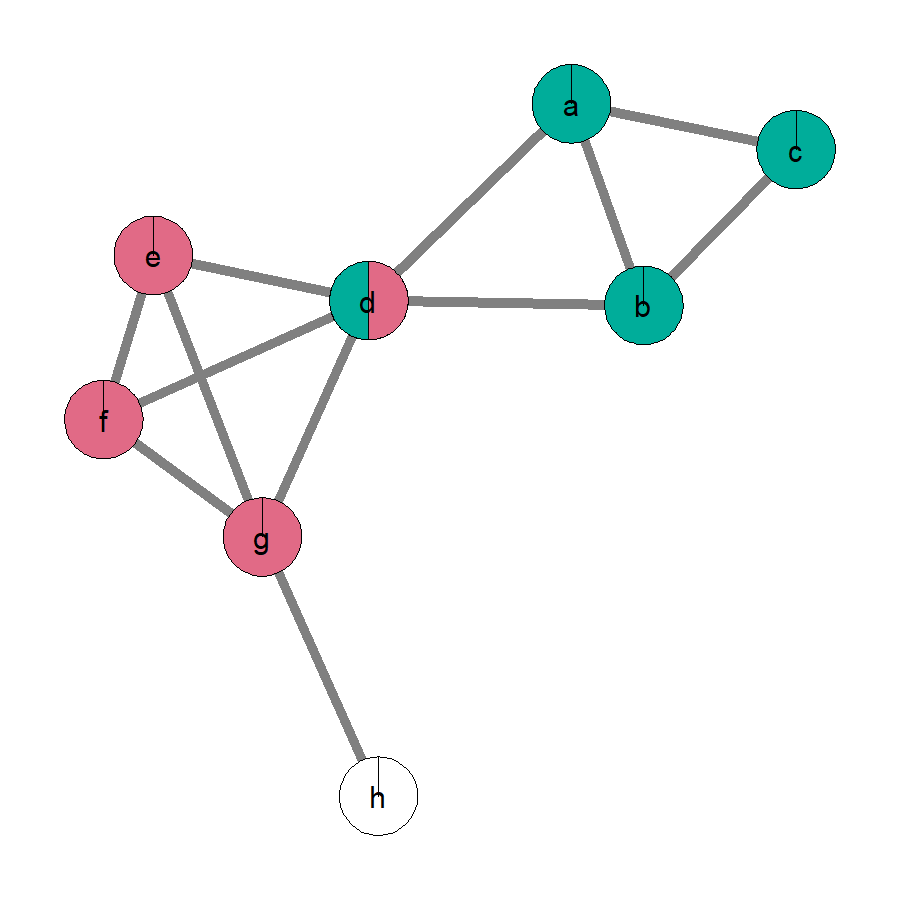Community partition by node coloring in unweighted network.

For weighted networks, the algorithm has just one intermediate additional step. Specifically, after identifying the $$k$$-cliques, they are considered further only if their Intensity exceeds a specified threshold $$I$$. The Intensity of a $$k$$-clique is defined as the geometric mean of the edge weights, namely

$I_C = \Bigg(\prod_{i<j;\,i,j\in C} w_{ij}\Bigg)^{2/k(k-1)}$ Where $$C$$ is the clique, $$i$$ and $$j$$ are the nodes, $$w$$ is the edge weight, and $$k$$ is the clique size. For instance, a 3-clique with edge weights 0.1, 0.2, and 0.3 would have

$I_C = (0.1*0.2*0.3)^{2/3(3-1)} \approx 0.18$

To show the influence of $$I$$, here is the network from the previous example, but this time I added edge weights.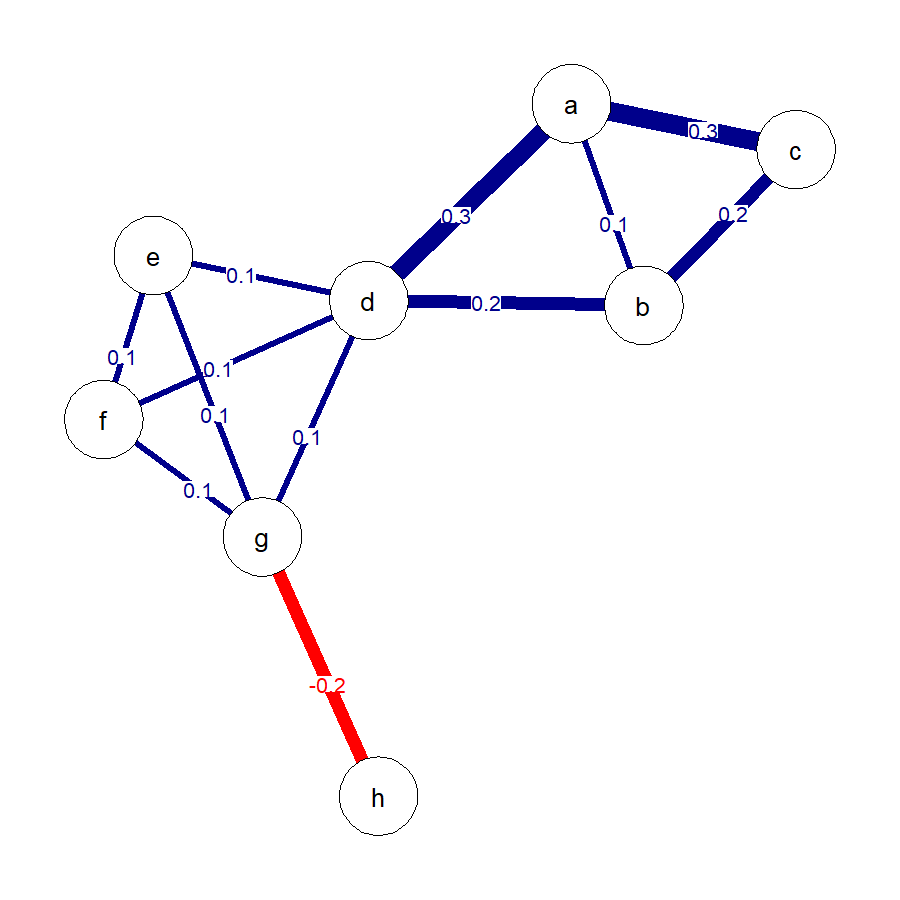Weighted network with eight nodes.

If $$I = 0.17$$, only two cliques ($$a$$$$b$$$$c$$, $$a$$$$b$$$$d$$) would survive the threshold. If only these are further considered, the clique percolation method would find only one community (the formerly green community) and four nodes would be isolated ($$e$$, $$f$$, $$g$$, $$h$$). If, however, $$I = 0.09$$, all cliques would survive the threshold, leading to the same community partition as for the unweighted network.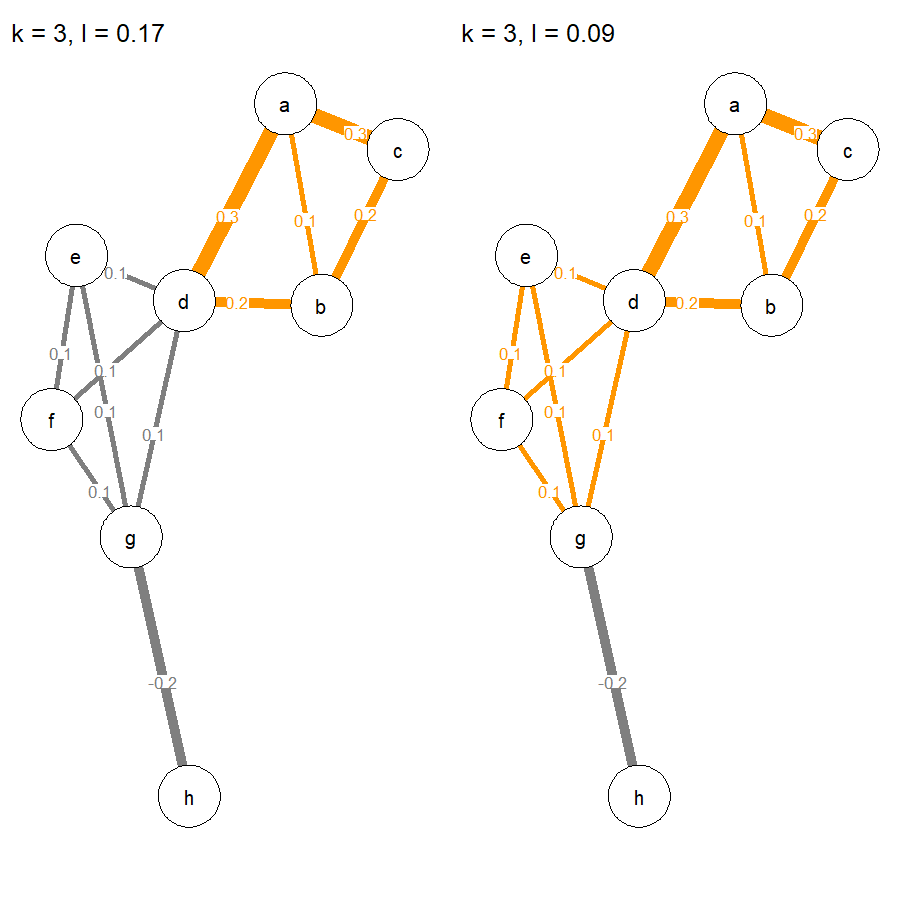Surviving cliques in weighted network depending on I.

The program that the developers of the clique percolation algorithm designed, CFinder, adds yet another intermediate step to this procedure, even though this intermediate step is not described in Farkas et al. (2007). Specifically, the Intensity threshold $$I$$ is applied not only to the $$k$$-cliques but additionally to the overlap of the $$k$$-cliques. For instance, in the case of the 3-cliques in our example, the overlap of the 3-cliques $$a$$$$b$$$$c$$ and $$a$$$$b$$$$d$$ is the edge $$a$$$$b$$. If $$I = 0.17$$, the two 3-cliques would survive the threshold. Subsequently, it is checked whether the $$a$$$$b$$ edge also exceeds the threshold. In the current case, it would not, because the edge weights is only 0.1. Given that the edge does not exceed the threshold, the two 3-cliques are not considered adjacent. Therefore, instead of putting them in the same community, the two 3-cliques are considered to be two separate communities with two shared nodes in CFinder.

### Optimizing k and I

It is challenging to optimize $$k$$ and $$I$$ for the clique percolation algorithm. For unweighted networks, only an optimal $$k$$ needs to be found, as $$I$$ is not used. Hence, when describing ways to optimize $$k$$ and $$I$$ for weighted networks, the same guidelines apply to unweighted networks, yet, only for $$k$$. Palla et al. (2005) and Farkas et al. (2007) provide guidelines based on ideas in percolation theory, which are better illustrated for weighted networks. Specifically, for each $$k$$, a large $$I$$ will lead to the exclusion of most if not all $$k$$-cliques for further consideration. The other extreme, a very low $$I$$, will lead to the inclusion of most if not all $$k$$-cliques for further consideration and these $$k$$-cliques then often combine to a gigantic component to which all nodes belong. The optimal $$I$$ for each $$k$$ is just above the point of the emergence of the gigantic component. Percolation theory supports that at this point, the size distribution of the communities follows a power-law. An indicator of this point with the broadest possible distribution is the ratio of the largest to second largest community sizes. $$I$$ and $$k$$ are optimal, if the largest community is twice as large as the second largest.

Notably, applying this threshold requires a large number of communities, otherwise this point might not be very stable. For somewhat smaller, yet still rather large networks, Farkas et al. (2007) propose to instead check the variance of the community sizes after excluding the largest community. The formula they provide is

$\chi = \sum^{n_\alpha \ne n_{max}} \frac{n_\alpha^2}{\big(\sum^\beta n_\beta\big)^2}$

where $$\chi$$ is the variance, $$n_\alpha$$ is a set of communities excluding the largest one, and $$n_\beta$$ is a set of communities that does neither include $$n_\alpha$$ nor the largest community. For instance, if there are four communities with 9, 7, 6, and 3 nodes, respectively, then one would exclude the largest community, and determine the variance of the last three by

$\chi = \frac{7^2}{(6+3)^2} + \frac{6^2}{(7+3)^2} + \frac{3^2}{7+6^2} \approx 1.02$

The logic behind $$\chi$$ is as follows. When $$I$$ is higher, there will be many equally small communities of size $$k$$. When $$I$$ is very low, there will be a gigantic community and many equally small ones. Thus, after excluding the largest community, for higher and smaller $$I$$, $$\chi$$ will be small. In contrast, when the community size distribution is broad (i.e., close to a power-law), then the variance of the communities (after excluding the largest community) will be higher. Thus, the point of the maximal variance is another way to optimize $$I$$ for different $$k$$.

Nevertheless, also a stable estimate of $$\chi$$ requires a moderate number of communities. If the network is very small, such that only a few communities can be expected, also the $$\chi$$ threshold is unreliable. For instance, networks that represent psychological constructs such as attitudes (Dalege et al., 2016) or emotions (Lange, Dalege, Borsboom, Kleef, & Fischer, 2020), entail typically only up to 25 nodes. Moreover, it would be undesirable to have, for instance, two communities of which one is twice as large as the other. Instead, equally sized communities are preferable. An alternative way to optimize $$I$$ for different $$k$$ for very small networks would be to rely on the entropy of the community partition, for instance, an entropy measure based on Shannon Information (Shannon, 1948). The idea is to find the most surprising community partition, defined as a low probability of knowing to which community a randomly picked node belongs. If there is only one community, surprisingness would be zero, because it would be certain that a randomly picked node belongs to this community. If there are, for instance, two communities of sizes 15 and 3, it would still not be very surprising, because most likely a randomly picked node will belong to the larger community. Surprisingness would be higher, however, when the communities are equal in size. Entropy based on Shannon Information captures this idea in the equation

$Entropy = -\sum_{i=1}^N p_i * \log_2 p_i$

where $$N$$ is the number of communities and $$p_i$$ is the probability of being in community $$i$$. For the four community sizes above (9, 7, 6, 3), the result would be

\begin{aligned} Entropy &= -\Bigg(\Big(\frac{9}{9+7+6+3} * log_2 \frac{9}{9+7+6+3}\Big) \\ & + \Big(\frac{7}{9+7+6+3} * log_2 \frac{7}{9+7+6+3}\Big) \\ & + \Big(\frac{6}{9+7+6+3} * log_2 \frac{6}{9+7+6+3}\Big) \\ & + \Big(\frac{3}{9+7+6+3} * log_2 \frac{3}{9+7+6+3}\Big)\Bigg) \\ &\approx 1.91 \end{aligned}

As pointed out, with only one community, this equation equals zero. When calculating entropy, isolated nodes are treated as another pseudo-community and shared nodes are split equally among the communities they belong to (e.g., a node shared by two communities is added as 0.5 nodes to each of them). As such, entropy favors equally sized communities with few isolated nodes in very small networks. This is exactly what would be desired. The $$I$$ that has the maximum entropy for the respective $$k$$ optimizes $$k$$ and $$I$$.

Because for very small networks, a specific amount of communities can occur just by chance alone, permutation tests may complement the calculation of entropy. Specifically, the edges of the network are randomly shuffled a number of times and for each of these random permutations, the highest entropy of a range of $$I$$ values is determined separately for each $$k$$. Over all permutations, this analysis leads to a distribution of entropy values for each $$k$$ separately. Only entropy values that exceed the confidence interval of the distribution of each $$k$$ can be considered more surprising than would already be expected by chance alone. For large and very large networks, the entropy threshold should, however, not be used. As it prefers equally sized community sizes, it would penalize the broad power-law distribution of community sizes that is preferred for larger networks.

More recently, Santiago et al. (Santiago, Soares, Quintero, & Jamieson, 2022) used simulations to compare the performance of different thresholds for optimizing $$k$$ and $$I$$. They argued convincingly that even entropy may often fail to detect the best community partition. Their simulations instead supported the conclusion that thresholds based on fuzzy modularity outperform entropy. Details are available in their manuscript.

Finally, for unweighted networks, the optimization of $$k$$ and $$I$$ is similar. Yet, given the limited range of possible $$k$$ (e.g., 3 to 6) many thresholds will not be very sensitive. Nevertheless, a small $$k$$ (e.g., $$k = 3$$) will often result in the emergence of a giant component to which most of the nodes belong. Increasing $$k$$ (e.g., $$k = 6$$) will often lead to a large number of smaller communities. $$k$$ is optimal when there is no giant component for very large networks, when $$\chi$$ is maximal for large networks, or when entropy is maximal for very small networks.

### Summary

In sum, the clique percolation algorithm proceeds by identifying $$k$$-cliques and by putting adjacent $$k$$-cliques (i.e., $$k$$-cliques that share $$k - 1$$ nodes) into a community. For weighted networks, the Intensity of the $$k$$-cliques must further exceed $$I$$. If not, they are removed from consideration. Finally, in the CFinder program, the $$k - 1$$ overlap of $$k$$-cliques must further exceed $$I$$. If not, the $$k$$-cliques are considered separately and not as a single community. Optimal $$k$$ and $$I$$ can be determined with the ratio test for large networks, $$\chi$$ for small networks, or entropy for very small networks.

## The workflow of the CliquePercolation package

The CliquePercolation package entails functions to conduct the clique percolation community detection algorithms for unweighted and weighted networks and to interpret the results. In what follows, an example is used to explain the workflow suggested by the package.

CliquePercolation accepts networks that were analyzed with the qgraph package (Epskamp, Cramer, Waldorp, Schmittmann, & Borsboom, 2012). qgraph accepts output from various different packages such as igraph (Csárdi & Nepusz, 2006), bootnet (Epskamp & Fried, 2018), or lavaan (Rosseel, 2012). For details see the documentation of qgraph. Moreover, I will use the Matrix (Bates & Maechler, 2019) package for the example below. Thus, we need to load the following packages in R to run the example

library(CliquePercolation) #version 0.3.0
library(qgraph)            #version 1.6.5
library(Matrix)            #version 1.2-18

First, I will create an example network. When you want to apply the clique percolation algorithm, you will already have a network. The network can be a qgraph object. Alternatively, you can also provide a symmetric matrix with rows and columns representing the nodes and each entry representing the edge connecting the respective nodes (i.e., an adjacency matrix or a weights matrix). The network I am using is weighted, with edge weights drawn from a random distribution with mean of 0.3 and a standard deviation of 0.1.

# create qgraph object; 150 nodes with letters as names; 1/7 of edges different from zero
W <- matrix(c(0), nrow = 150, ncol = 150, byrow = TRUE)
name.vector <- paste(letters[rep(seq(from = 1, to = 26), each = 26)],
letters[seq(from = 1, to = 26)], sep = "")[1:nrow(W)]
rownames(W) <- name.vector
colnames(W) <- name.vector

set.seed(4186)
W[upper.tri(W)] <- sample(c(rep(0,6),1), length(W[upper.tri(W)]), replace = TRUE)
rand_w <- stats::rnorm(length(which(W == 1)), mean = 0.3, sd = 0.1)
W[which(W == 1)] <- rand_w

W <- Matrix::forceSymmetric(W)
W <- qgraph::qgraph(W, theme = "colorblind", layout = "spring", cut = 0.4)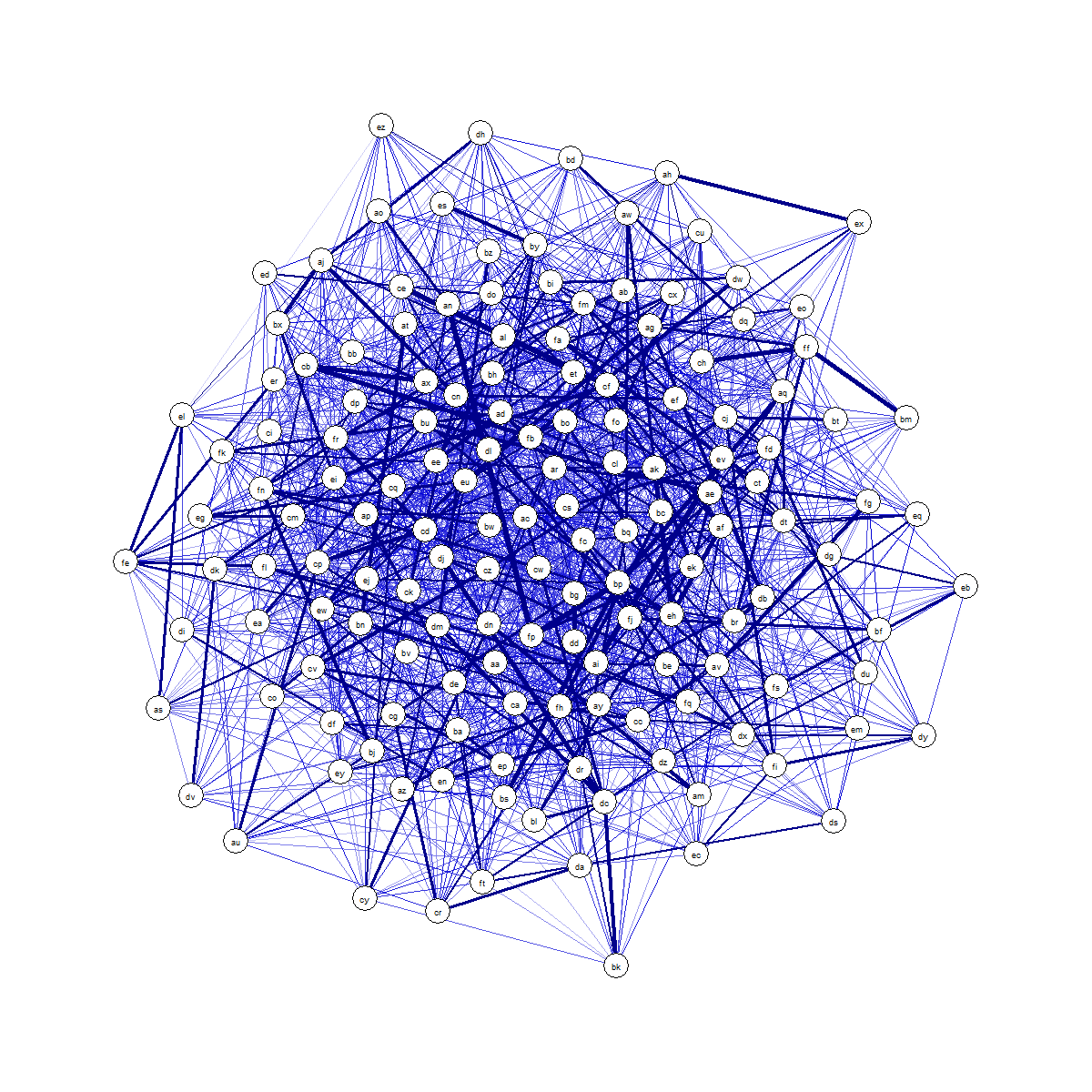Weighted network with 150 nodes.

To run the clique percolation algorithm for weighted networks, we initially need to optimize $$k$$ and $$I$$. To this end, the cpThreshold function can be used. For a specific network (i.e., a qgraph object or a matrix), it determines the ratio of the largest to second largest community, $$\chi$$, entropy and/or (signed) fuzzy modularity over a range of $$k$$ and $$I$$ values. It does so by running the clique percolation algorithm for either unweighted (method = "unweighted") or weighted networks (method = "weighted"). The algorithm as implemented in CFinder can also be requested (method = "weighted.CFinder"). For each $$k$$ and $$I$$ combination, cpThreshold determines the respective threshold and saves the results in a data frame, specifying the respective $$k$$, $$I$$, the number of communities, the number of isolated nodes, and the requested thresholds. Note that the ratio threshold can be determined only when there are at least two communities and $$\chi$$ can be determined only when there are at least three communities. Otherwise, the function will return NA values in these cases. Entropy and (signed) fuzzy modularity can always be determined. In the current case, as the network is rather large, I will request only the ratio and $$\chi$$ thresholds. I will do so for $$k$$ values of 3 and 4 and $$I$$ values of 0.40 to 0.01 in steps of 0.005. Even smaller steps might in many cases be preferred, given that even small changes of 0.005 can lead to rather different (and potentially optimal) values. However, to save computation time, I took these larger steps. The range of $$I$$ values was chosen based on the fact that my mean edge weight was set to 0.3 when generating the network. Thus, $$I = 0.40$$ should allow me to find a broad community size distribution. However, to be sure, one could start by setting the highest tested value of $$I$$ to the maximum edge weight in the network. This is recommended by Farkas et al. (2007). But then, very high $$I$$ values will rarely lead to the identification of any $$k$$-clique. The k.range and I.range are entered as vectors of numbers. The requested thresholds are also entered as a vector of strings. Depending on the network, this function may need lots of time to run (the code below ran for 18 minutes on my laptop). To see how long it takes, a progress bar is implemented.

The cpThreshold function is used as follows

thresholds <- cpThreshold(W, method = "weighted", k.range = c(3,4),
I.range = c(seq(0.40, 0.01, by = -0.005)),
threshold = c("largest.components.ratio","chi"))

We can now access the data frame that is saved in the object thresholds

thresholds

To decide in favor of optimal $$I$$ values for each $$k$$, we now check at which $$I$$ the ratio threshold is jumping abruptly to a high level, thereby crossing the ratio 2. The $$I$$ just before the jump should ideally also be the point of the highest $$\chi$$. For $$k = 3$$, the ratio first crossed 2 at $$I = 0.35$$ with $$\chi$$ being rather large. This solution has 45 communities and nine isolated nodes. For $$k = 4$$, the ratio first crossed 2 at $$I = 0.27$$. The $$\chi$$ is not very large and subsequently the nodes never reach a stage at which they are all part of a single community, most likely because $$k$$ is too large to allow that to happen. This already indicates that $$k = 4$$ is probably too high. This solution has 62 communities and 29 isolated nodes. Hence, also the number of isolated nodes is rather high. Still, we can now use these optimized values to run the clique percolation method. For this, we apply the cpAlgorithm function. Specifically, the cpAlgorithm function takes a network (i.e., a qgraph object or a matrix) and runs the clique percolation algorithm for unweighted (method = "unweighted") or weighted networks (method = "weighted"). The algorithm as implemented in CFinder can also be requested (method = "weighted.CFinder"). Additionally, we enter the optimal $$k$$ and $$I$$ values determined via cpThreshold. Thus, we run cpAlgorithm twice, namely

cpk3I.35 <- cpAlgorithm(W, k = 3, method = "weighted", I = 0.35)
cpk4I.27 <- cpAlgorithm(W, k = 4, method = "weighted", I = 0.27)

These objects have the class cpAlgorithm. They are lists, entailing the results of the clique percolation algorithm. When printing the objects in the console, they offer a brief summary of the results. For instance

cpk3I.35
#>
#> Results of clique percolation community detection algorithm
#>
#> --------------------
#>
#> User-specified Settings
#>
#> method = weighted
#> k = 3
#> I = 0.35
#>
#> --------------------
#>
#> Results
#>
#> Number of communities: 45
#> Number of shared nodes: 94
#> Number of isolated nodes: 9
#>
#> --------------------
#>
#> For details, use summary() (see ?summary.cpAlgorithm).

Using summary, it is possible to get more detailed information about the communities, shared nodes, and isolated nodes.

summary(cpk3I.35)

By default, this function will present the communities, shared nodes, and isolated nodes with labels as identifies of the nodes. It is also possible to use the numbers as identifiers of the nodes or to restrict the output. For instance, when only the shared nodes with numbers as identifies of nodes should be requested

summary(cpk3I.35, details = "shared.nodes.numbers")

Alternatively, it is possible to access all information directly from the objects. Each element can be requested via the $ operator. For instance, the list of communities with the node numbers as identifiers of the nodes for the $$k = 3$$ solution can be requested by typing cpk3I.35$list.of.communities.numbers

As an example, community 45 entails nodes 9, 12, and 33. The same results with the labeled nodes can be requested via

cpk3I.35$list.of.communities.labels The nodes 9, 12, and 33 in community 45 are named “ai”, “al”, and “bg”. To decide in favor of one solution, we can investigate the community size distribution. This should be maximally broad, similar to a power-law. To look at the community size distribution, we can use another function, namely cpCommunitySizeDistribution. It takes the list of communities generated by cpAlgorithm and turns it into a distribution plot. As the plot may not please you visually, the function also returns a data frame with the frequency data if you assign the results to an object. Thus, you are free to plot these data in any way you want. The default line color of the distribution is red, but could be changed via the color.line argument. cpCommunitySizeDistribution(cpk3I.35$list.of.communities.numbers)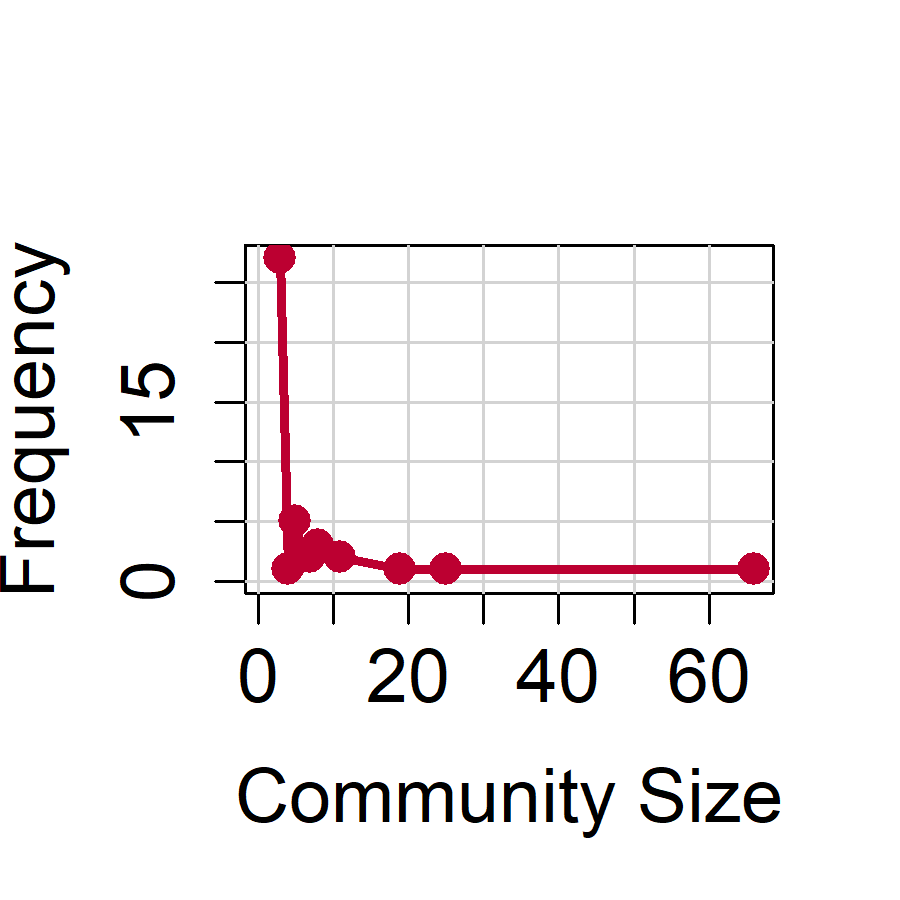Community size distribution with k = 3 and I = 0.35.

cpCommunitySizeDistribution(cpk4I.27$list.of.communities.numbers)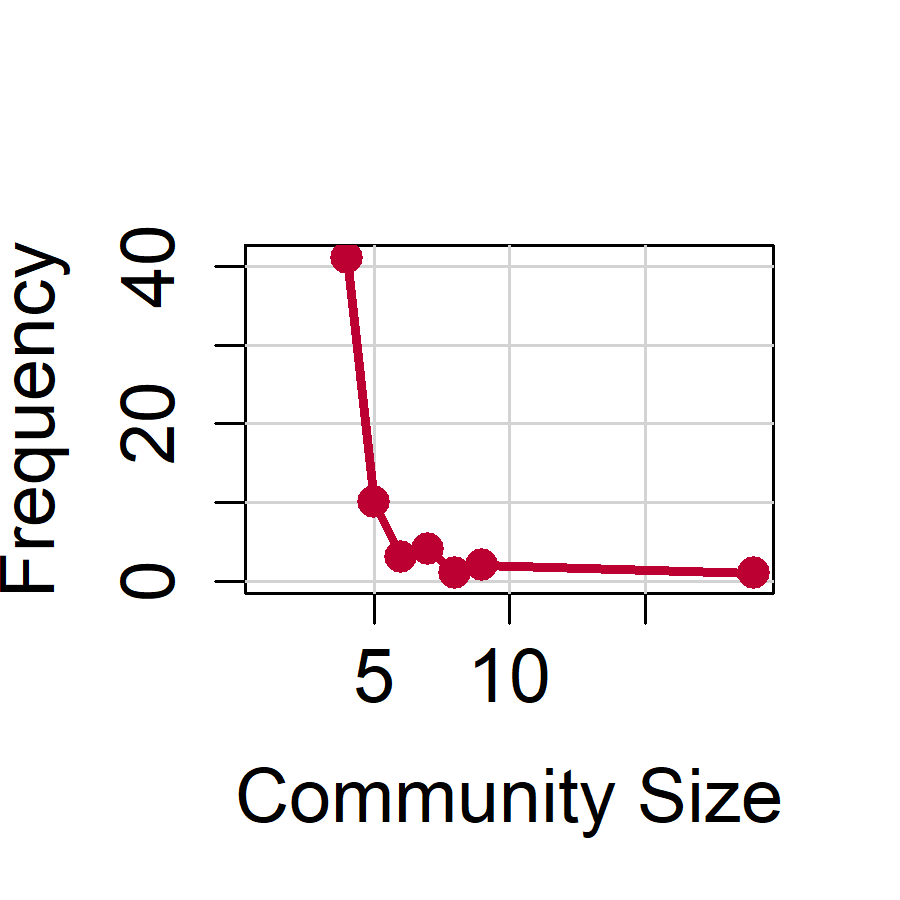Community size distribution with k = 4 and I = 0.27. Both distributions look very much like a power-law, given that multiple very large community sizes occur rarely. To actually test whether the distribution for $$k = 3$$ fits a power-law, cpCommunitySizeDistribution has another argument, test.power.law. It tests the fit via the fit_power_law function of the igraph package (Csárdi & Nepusz, 2006). The following code runs the test fit_pl_k3I.35 <- cpCommunitySizeDistribution(cpk3I.35$list.of.communities.numbers, test.power.law = TRUE)

The object fit_pl_k3I.35 now includes the output of fit_power_law, which can be accessed via

fit_pl_k3I.35$fit.power.law #>$continuous
#>  FALSE
#>
#> $alpha #>  2.72733 #> #>$xmin
#>  3
#>
#> $logLik #>  -88.19429 #> #>$KS.stat
#>  0.1599964
#>
#> $KS.p #>  0.1995392 The Kolmogorov-Smirnov Test is not significant, $$p = .20$$, indicating that the hypothesis of a power-law cannot be rejected. This finding is in line with the notion that the distribution follows a power-law with $$\alpha = 2.73$$. Thus, we decide in favor of $$k = 3$$ and $$I = .35$$ as the optimal parameters for clique percolation. Oftentimes, researchers are interested in plotting the solution of the clique percolation algorithm in another network, such that a node represents a community, and the edges between nodes represent the number of nodes that two communities share (i.e., the community graph). Plotting this network can be done with the cpCommunityGraph function. It also takes the list of communities from cpAlgorithm and turns it into the community network. To showcase which node represents which network, the node sizes can be plotted proportional to the largest node (node.size.method = "proportional"). To do this, we set the size of the largest node via max.node.size and the others are scaled accordingly. If the proportional visualization is not preferred, we can also plot all nodes with equal size (node.size.method = "normal"). As the community network is plotted via qgraph, one can also add all other arguments available in qgraph to make the graph more pleasing. Moreover, next to plotting the community network, the function also returns the weights matrix of the community graph if the results are assigned to an object. This matrix could then be used for other purposes. The current community network can be plotted as follows, by additionally using a spatial arrangement and edge coloring from qgraph. commnetwork <- cpCommunityGraph(cpk3I.35$list.of.communities.numbers,
node.size.method = "proportional",
max.node.size = 20,
theme = "colorblind", layout = "spring", repulsion = 0.4)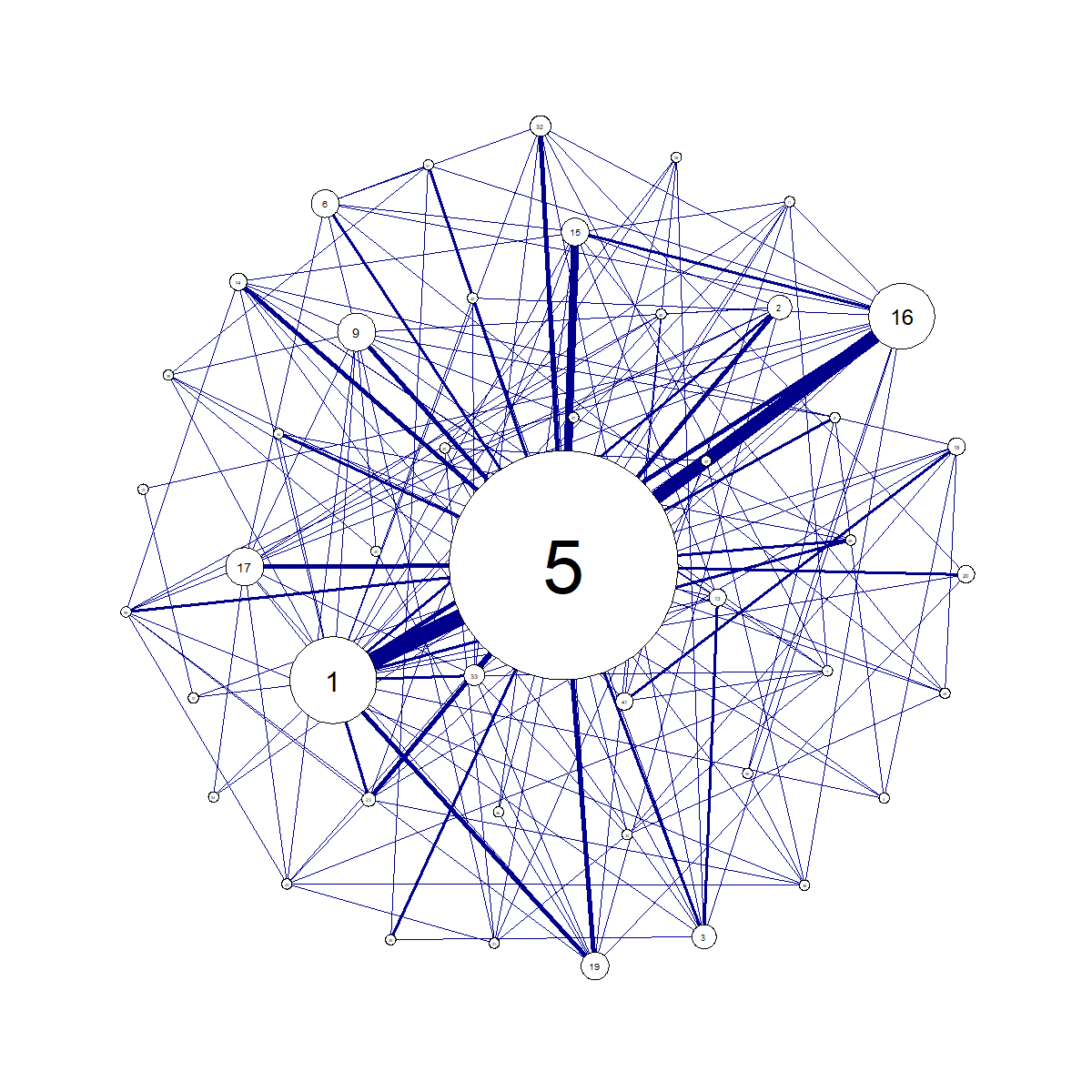Community network with k = 3 and I = 0.35.

The weights matrix can be accessed via

commnetwork$community.weights.matrix As was already clear from the community size distribution, most communities are rather small, while community 5 is very large. Yet there is overlap among many communities, that, in the case of an actual network, should then be interpreted thematically. ### Very small networks For very small networks, the above procedures may not all apply. First, the ratio and $$\chi$$ thresholds cannot be determined or are not very informative for very small networks. Second, a community network may not be the most informative representation of the results, if there are only very few communities to begin with. To showcase the somewhat altered workflow with very small networks, we will use a very small, unweighted network. W <- matrix(c(0,1,1,1,0,0,0,0,0,0, 0,0,1,1,0,0,0,0,0,0, 0,0,0,0,0,0,0,0,0,0, 0,0,0,0,1,1,0,0,0,0, 0,0,0,0,0,1,0,0,0,0, 0,0,0,0,0,0,1,1,1,0, 0,0,0,0,0,0,0,1,1,0, 0,0,0,0,0,0,0,0,1,0, 0,0,0,0,0,0,0,0,0,1, 0,0,0,0,0,0,0,0,0,0), nrow = 10, ncol = 10, byrow = TRUE) W <- forceSymmetric(W) rownames(W) <- letters[seq(from = 1, to = nrow(W))] colnames(W) <- letters[seq(from = 1, to = nrow(W))] W <- qgraph(W, edge.width = 4)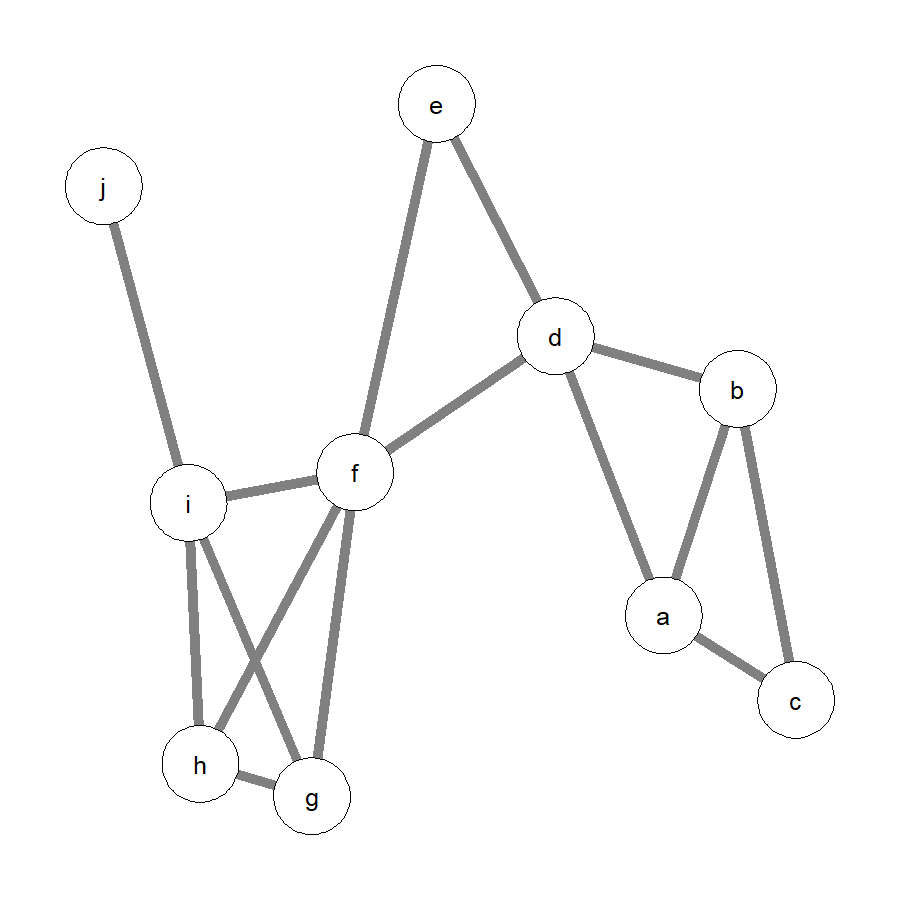Unweighted network with ten nodes. Again, we will first use cpThreshold. As the network is unweighted, we need to specify only the k.range but not the I.range. As the network is very small, we will request entropy instead of the ratio and $$\chi$$. thresholds.small <- cpThreshold(W, method = "unweighted", k.range = c(3,4), threshold = "entropy") You can now access the data frame that is saved in the object thresholds.small thresholds.small #> k Number.of.Communities Number.of.Isolated.Nodes Entropy.Threshold #> 1 3 3 1 1.8567796 #> 2 4 1 6 0.9709506 I display it here because, as compared to the previous example, it is short. For $$k = 3$$, there are three communities and one isolated node with an entropy of 1.86. For $$k = 4$$, there is one community and six isolated nodes with an entropy of 0.97. Now, we can estimate whether this entropy is higher than would already be expected by chance. The permutation test for very small networks is implemented in the function cpPermuteEntropy. For a specific network (i.e., a qgraph object or a matrix) and a cpThreshold object, cpPermuteEntropy creates n permutations of the network, performs cpThreshold for each, extracts the highest entropy for each $$k$$, and determines the confidence interval of the entropy for each $$k$$. n = 100 and the 95% confidence interval are the default settings. Larger n are certainly desired but can lead to large computation time. A progress bar again indicates how long it will take. Given that permutations are random, I will set a seed to allow reproducing the results. As the function relies on parallel computation, it is not possible to set the seed outside the function. Instead, it is necessary to set the seed argument inside the function. By default, the function uses half of the computational power of the computer, but it is possible to set the number of used cores with the ncores argument. The function can be used as follows permute <- cpPermuteEntropy(W, cpThreshold.object = thresholds.small, n = 100, interval = 0.95, ncores = 2, seed = 4186) #> Permutating... The resulting object has the class cpPermuteEntropy. The printing function shows the results, following a repetition of the specified settings. First, it shows the confidence intervals for each $$k$$. Second, the function also takes the original cpThreshold object specified in cpThreshold.object and removes all rows that do not exceed the upper bound of the confidence interval. permute #> #> Confidence intervals for entropy values of random permutations of original network #> #> -------------------- #> #> User-specified Settings #> #> n = 100 #> interval = 0.95 #> CFinder = FALSE #> ncores = 2 #> seed = 4186 #> #> #> -------------------- #> #> Confidence intervals #> #> k 95% CI lower 95% CI upper #> 3 1.152 1.276 #> 4 0.086 0.227 #> #> #> -------------------- #> #> Extracted rows from cpThreshold object #> #> k Number.of.Communities Number.of.Isolated.Nodes Entropy.Threshold #> 3 3 1 1.857 #> 4 1 6 0.971 The resulting object is also a list with the two respective objects, accessible via permute$Confidence.Interval
permute$Extracted.Rows In the current example, both solutions, for $$k = 3$$ and $$k = 4$$, exceed the upper bound of the confidence interval and are thus still in the data frame. Now we can choose an optimal $$k$$. Even though both exceed the upper bound of the confidence interval, the number of isolated nodes for $$k = 4$$ is rather high, leading us to accept $$k = 3$$ as the optimal $$k$$. Using this optimal $$k$$, we run the cpAlgorithm cpk3 <- cpAlgorithm(W, k = 3, method = "unweighted") By inspecting the results with cpk3 #> #> Results of clique percolation community detection algorithm #> #> -------------------- #> #> User-specified Settings #> #> method = unweighted #> k = 3 #> #> -------------------- #> #> Results #> #> Number of communities: 3 #> Number of shared nodes: 2 #> Number of isolated nodes: 1 #> #> -------------------- #> #> For details, use summary() (see ?summary.cpAlgorithm). summary(cpk3) #> #> -------------------- #> Communities (labels as identifiers of nodes) #> -------------------- #> #> Community 1 : f g h i #> Community 2 : d e f #> Community 3 : a b c d #> #> #> -------------------- #> Shared nodes (labels as identifiers of nodes) #> -------------------- #> #> d f #> #> #> -------------------- #> Isolated nodes (labels as identifiers of nodes) #> -------------------- #> #> j we see that we indeed have three communities, one entailing nodes $$a$$, $$b$$, $$c$$, and $$d$$, one entailing nodes $$d$$, $$e$$, and $$f$$, and one entailing nodes $$f$$, $$g$$, $$h$$, and $$i$$. We further see that nodes $$d$$ and $$f$$ are shared nodes and that node $$j$$ is isolated. We could have also requested the fuzzymod threshold for such a small network using thresholds.small.fuzzymod <- cpThreshold(W, method = "unweighted", k.range = c(3,4), threshold = c("entropy","fuzzymod")) We can again inspect the results with thresholds.small.fuzzymod #> k Number.of.Communities Number.of.Isolated.Nodes Entropy.Threshold FuzzyMod #> 1 3 3 1 1.8567796 0.3211111 #> 2 4 1 6 0.9709506 0.1033333 showing that fuzzy modularity favors the same $$k$$ and $$I$$. One advantage, however, is that we do not need to run any permuation test for fuzzy modularity. Finally, we would like to plot the results of the clique percolation algorithm. However, using the community graph for a network with only three communities would not be very informative. In the current case, the community network would have three nodes with two edges. For very small networks, an alternative may be reasonable, namely plotting the community partition directly on the original network that was analyzed. This can be achieved with the cpColoredGraph function. It takes the community partition from cpAlgorithm and assigns qualitatively different colors to the communities with the help of the colorspace package (Zeileis et al., 2019). It is based on the HCL color space, generating palettes of colors that are rather balanced and visually pleasing. Isolated nodes will be displayed white. Shared nodes will be divided in multiple colors, one for each community they belong to. Original qgraph arguments can be used to make the network appear as before. colored.net1 <- cpColoredGraph(W, list.of.communities = cpk3$list.of.communities.labels,
edge.width = 4)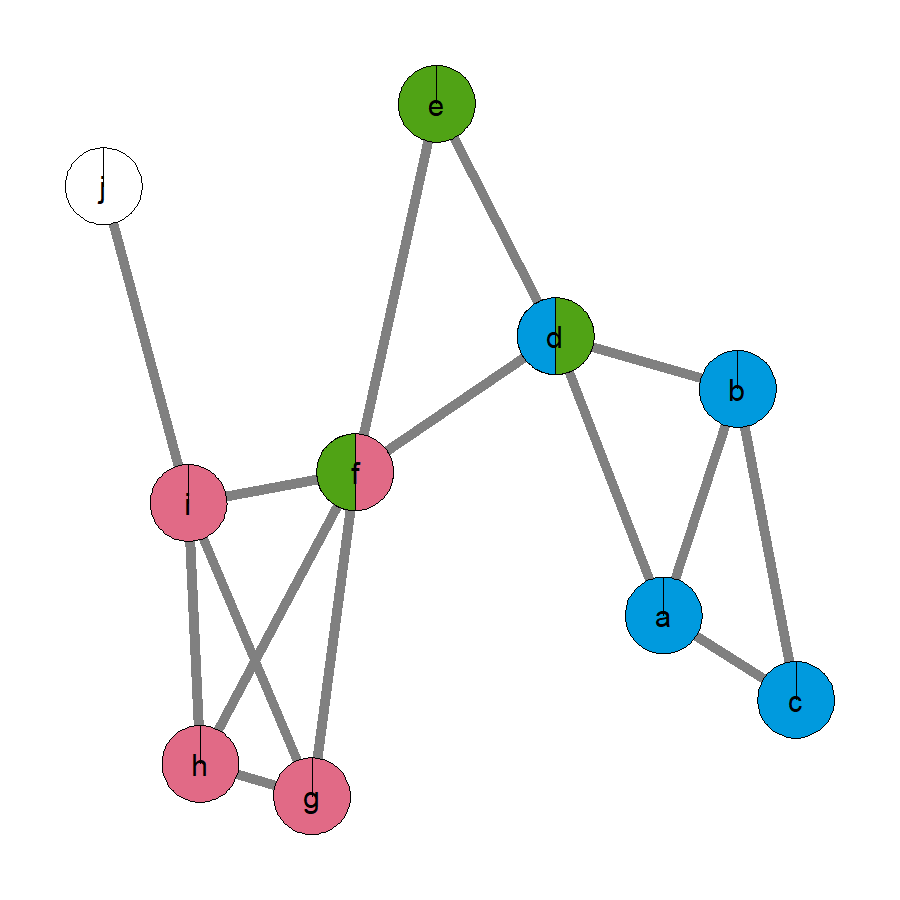Community coloring I.

The function also returns the colors assigned to the communities and the colors assigned to the nodes, if the results are assigned to an object. The colors assigned to the nodes are a list with vectors for each node. If a node is shared, all its colors are in the vector. These results can be accessed by

colored.net1$colors.communities colored.net1$colors.nodes

The function used for generating qualitatively different colors in the package colorspace is called qualitative_hcl. It generates colors of a range of hue values, holding chroma and luminance constant. cpColoredGraph uses the recommended defaults from qualitative_hcl. However, these defaults can be overwritten, if different colors are desired. For instance, to plot the graph from before with colors in the range from yellow to blue, lower chroma, and higher luminance, the following code could be used

colored.net2 <- cpColoredGraph(W, list.of.communities = cpk3$list.of.communities.labels, h.cp = c(50, 210), c.cp = 70, l.cp = 70, edge.width = 4)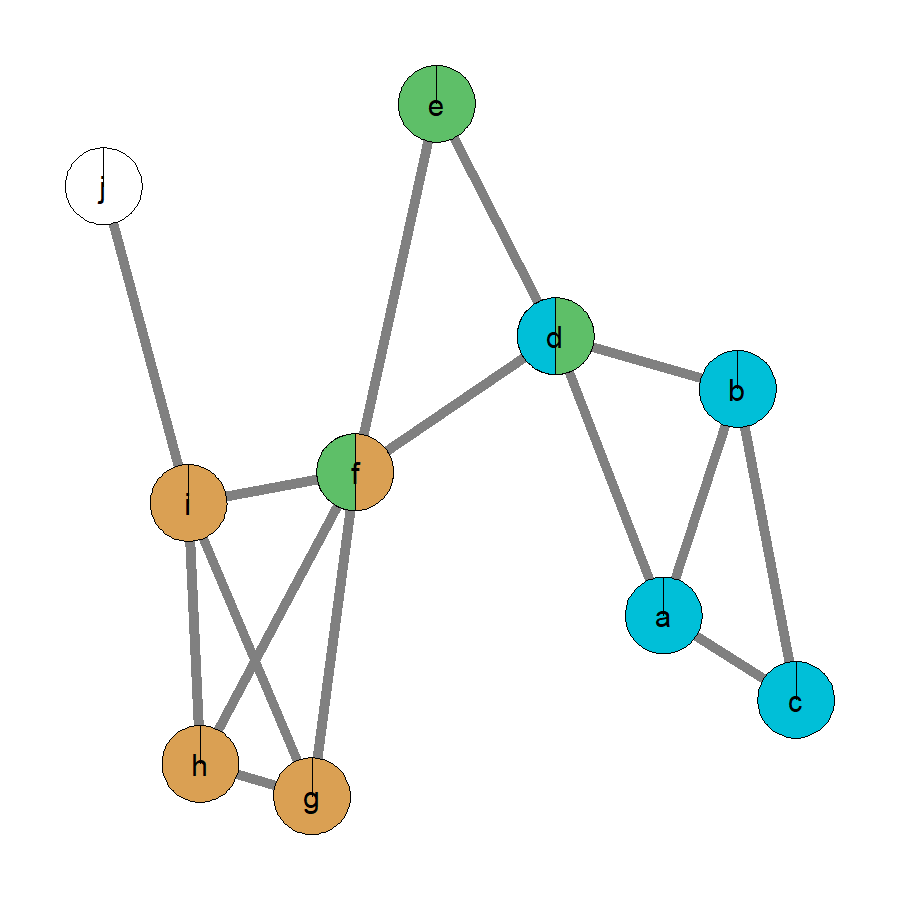Community coloring II. Oftentimes, the clique percolation algorithm is probably run on a network, for which there are predefined sets of nodes. For instance, it could be that in the example network, nodes $$a$$, $$b$$, $$c$$, $$d$$, $$e$$, and $$f$$ are from one set (e.g., one questionnaire) and nodes $$g$$, $$h$$, $$i$$, and $$j$$ are from another set (e.g., another questionnaire). Such sets of nodes can be taken into account, when plotting the network. For this, the list.of.sets argument needs to be specified, a list of vectors in which each vector codes one set of nodes. If this is done, cpColoredGraph assigns qualitatively different colors to the sets. Then, it checks whether the identified communities are pure (i.e., they entail nodes from only one set) or they are mixed (i.e., they entail nodes from multiple sets). For pure communities of each respective set, it takes the assigned color and fades it toward white, using colorspace’s sequential_hcl. It does so such that there are as many non-white colors as there are pure communities of a set. Larger communities will be assigned stronger colors. If a community is mixed with nodes from different sets, the colors of these sets will be mixed proportionally to the number of nodes from each set. For instance, if a community entails two nodes from one set and one node from another set, the colors will be mixed 2:1. For this, the colors are mixed subtractively (how paint mixes), which is difficult to implement. The mixing is done with an algorithm taken from Scott Burns (see http://scottburns.us/subtractive-color-mixture/ and http://scottburns.us/fast-rgb-to-spectrum-conversion-for-reflectances/). In short, colors are translated into reflectance curves. This is achieved by taking optimized reflectance curves of red, green, and blue and integrating them according to the RGB value of the color. The reflectance curves of the colors that have to be mixed are averaged via the weighted geometric mean. The resulting reflectance curve is translated back to RGB. According to substantive tests performed by Scott Burns, the mixing works nicely and is computationally efficient. Yet, it may not always produce precise results. In the current example, with the list of sets mentioned above, two communities will be pure ($$a$$$$b$$$$c$$$$d$$, $$d$$$$e$$$$f$$) and one will be mixed ($$f$$$$g$$$$h$$$$i$$). The two pure communities will hence be faded from the assigned colored towards white, making the smaller community ($$d$$$$e$$$$f$$) lighter. The mixed community will be mixed in 3:1, as there are three nodes from one set and one node from the other set. This could be achieved with the following code (using the first coloring). #define list.of.sets list.of.sets1 <- list(c("a","b","c","d","e","f"), c("g","h","i","j")) colored.net3 <- cpColoredGraph(W, list.of.communities = cpk3$list.of.communities.labels,
list.of.sets = list.of.sets1,
edge.width = 4)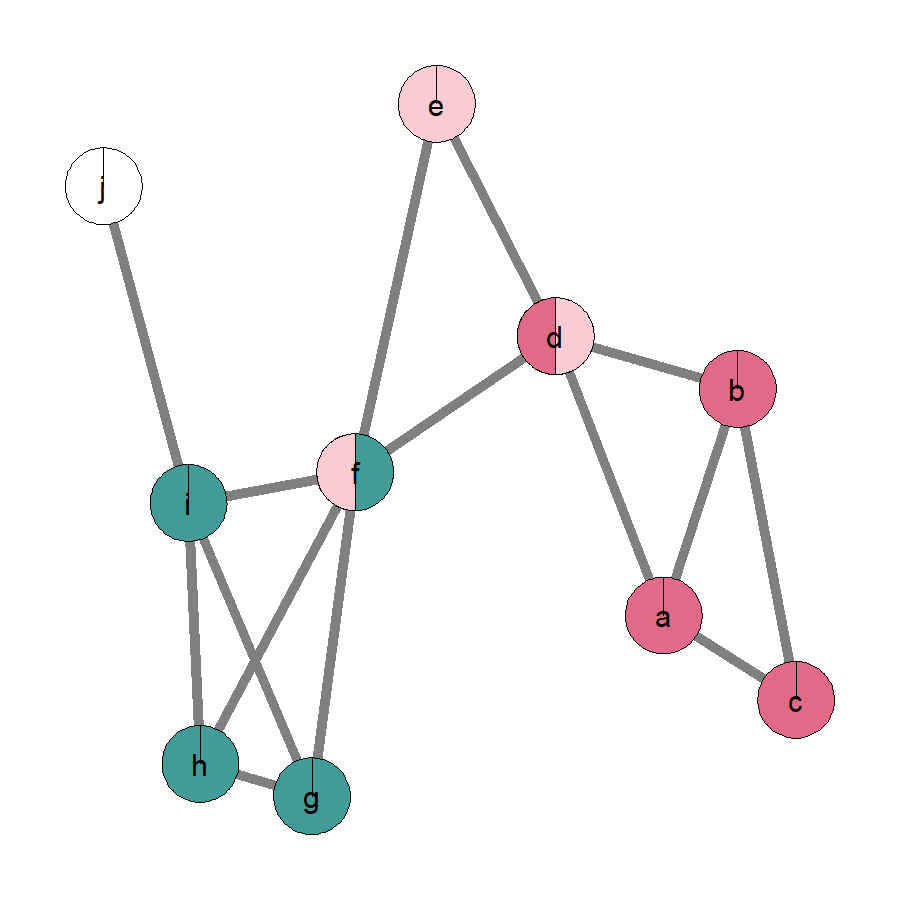Community coloring III.

Now you can access another element, that cpColoredGraph returns, namely a vector with the colors assigned to the sets.

colored.net3$basic.colors.sets Note that the colors are not identical to the colors in the network, as they were faded for two communities and mixed for another community. The actual colors assigned to the sets look like thisColor patches. The community entailing $$f$$, $$g$$, $$h$$, and $$i$$ is a mix of both colors. Moreover, the fading for pure communities always generates the faded palette for the number of pure communities of a set plus 1 (the plus one prevents that one community becomes white, which is reserved for isolated nodes). As such, if there are different numbers of pure communities for different sets in a network, or if across different networks there are different numbers of pure communities for the same set, their luminance values are not directly comparable. To make them comparable, we can assign a scale to the fading, such that we specify how many faded colors the set palettes should have. This can be achieved by specifying the set.palettes.size argument. For instance, if it is set to 5, then for all pure communities of all sets, cpColoredGraph will generate five faded colors. Larger communities will get the stronger colors asf. As such, if there are more pure communities of one set than for another set in the same network, there will be lighter communities for the set with more than with less pure communities. Moreover, if one set of nodes produces more communities in one network than in another, its nodes will overall be lighter in the network in which it produced more communities. In our example network, there were two pure communities of one set. Per default cpColoredGraph generated two faded non-white colors and assigned them to the communities. Thus, one community is rather strongly colored and the other is almost white. This overestimates that they are rather similar in size. Scaling them such that all pure communities are assigned colors from a set of five colors can be achieved as follows colored.net4 <- cpColoredGraph(W, list.of.communities = cpk3$list.of.communities.labels,
list.of.sets = list.of.sets1, set.palettes.size = 5,
edge.width = 4)